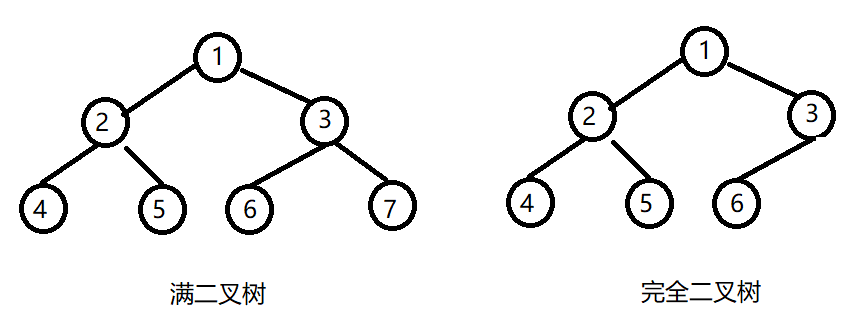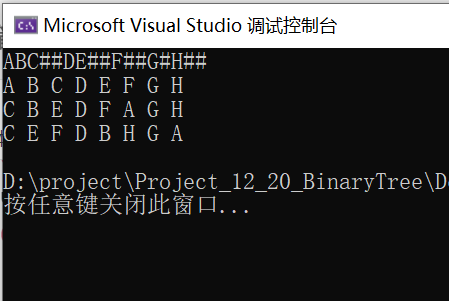• 1、根据输入的数据建立一个二叉树； 2、分别采用前序、中序、后序的遍历方式显示输出二叉树的遍历结果 3、采用非递归的编程方法，分别统计二叉树的节点个数、度为1、度为2和叶子节点的个数，以及数据值的最大值和...
• 5/27/2020 数据结构课程设计报告 数据结构课程设计报告 大学 大学 XXX XXX 电子与信息工程学院 电子与信息工程学院 数据结构课程设计报告 数据结构课程设计报告 年度第一学期 年度第一学期
• cout二叉树链表存储功能演示 "; cout; cout第一种输入法：默认广义表 "; cout第二种输入法：键盘输入广义表 "; cout第三种输入法：新建树根(逐个输入)"; cout增加儿子数据 "; cout删除叶子结点或仅仅根 "; ...
• /*实验要求 1按先序序列构造一棵二叉链表表示的二叉树T 2对这棵二叉树进行递归遍历先序中序后序以及层次遍历遍历序列分别输出结点的遍历序列 3. 对这棵树用非递归方式进行遍历先序中序以及后序遍历序列分别输出结点...
• 主要介绍了实现二叉树的创建（先序）、递归及非递归的先、中、后序遍历
• 实现了基本二叉树算法，利用链表实现，计算树高，插入删除等
• 教育资料 实验三 二叉树基本操作 学院:物理与电子学院 班级:电信1105班 姓名:刘岩 学号:1404110729 一实验目的 1熟悉二叉树基本操作掌握二叉树的实现以及实际应用 3加深对于二叉树的理解逐步培养解决实际问题的...
• 5/27/2020 244305643.doc 244305643.doc 数据结构与数据库 数据结构与数据库 实验报告 实验报告 实验题目 实验题目 二叉树基本操作及运算 二叉树基本操作及运算
• //使用二叉链表方式定义二叉树结构 typedef char ElemType; typedef struct BtNode { BtNode* leftchild;//左孩子 BtNode* rightchild;//右孩子 ElemType data;//数据域 }BtNode,*BinaryTree; ...
1.树的基本概念(1) 节点的度：一个节点含有的子树个数

例：图中A节点的度为1，D节点的度为2

(2) 树的度：一棵树中，最大的节点的度为树的度

例：图中二叉树的度为2

(3) 叶节点：也称为终端节点，度为零的节点

例：图中二叉树的叶节点有C、G、F

(4) 分支节点：也称为非终端节点，度不为零的节点

例：图中二叉树的分支节点为A、B、D、E

(5) 节点的层次：从根开始定义起，根为第一层，根的子节点为第二层，以此类推。

例：图中二叉树的层次为5层

（6）深度：对于任意节点n,n的深度为从根到n的唯一路径长。

例：图中二叉树的深度为5

（7）高度：对于任意节点n,n的高度为从n到一片树叶的最长路径长。 例：图中C节点的高度为：C->B->A，即2

2.二叉树的基本概念

（1）若二叉树的层次从0开始，则在二叉树的第i层最多有2i个节点

（2）高度为k的二叉树最多有[2^(k+1)]-1个节点

（3）如果二叉树叶结点个数为n0,度为2的非叶节点个数为n2,则有n0=n2+1

（4）满二叉树：每一层的节点达到其最大值

（5）完全二叉树：若二叉树高度为h，则共有h+1层，除第h层外，其他各层的节点数都达到最大个数，第h层从右向左连续缺若干节点 *具有n个节点的完全二叉树的高度为[log(n+1)]-13.二叉树基本操作
//1.创建节点
#include<iostream>
using namespace std;
#define END "#"//结束标志
//使用二叉链表方式定义二叉树结构
typedef char ElemType;
typedef struct BtNode
{
BtNode* leftchild;//左孩子
BtNode* rightchild;//右孩子
ElemType data;//数据域
}BtNode,*BinaryTree;

//2.创建二叉树
//递归创建
{
BtNode* s=(BtNode*)malloc(sizeof(BtNode*));
if(NULL==s) exit(EXIT_FAILURE);
memset(s,0,sizeof(BtNode*));//清零
return s;
}
BtNode* CreateTree()
{
ElemType ch;
BtNode* s = NULL;
cin >> ch;
if(ch != END)
{
s->data=ch;
s->leftchild=CreateTree();
s->rightchild=CreateTree();
}
return s;
}
//中序后序创建二叉树
int FindIs(char* is,char value,int n)
{
int pos=-1;
for(int i=0;i<n;i++)
{
if(is[i]==value)
{
pos=i;
break;
}
}
return pos;
}
BtNode* CreateILTree(char* is,char* ls,int n)
{
BtNode* s=NULL;
if(is!=NULL && ls!=NULL && n>0)
{
s->data=ls[n-1];//后序的最后一个数为二叉树根节点
int pos=FindIs(is,ls[n-1],n);
if(pos==-1) exit(EXIT_FAILURE);
s->leftchild=CreateILTree(is,ls,pos);
s->rightchild=CreateILTree(is+pos+1,ls+pos,n-pos-1);
}
return s;
}

//3.递归遍历二叉树
//先序遍历
void PreOrder(BtNode* ptr)
{
if(ptr!=NULL)
{
cout<<ptr->data<<" ";
PreOrder(ptr->leftchild);
PreOrder(ptr->rightchild);
}
}
//中序遍历
void InOrder(BtNode* ptr)
{
if(ptr!=NULL)
{
InOrder(ptr->leftchild);
cout<<ptr->data<<" ";
InOrder(ptr->rightchild);
}
}
//后序遍历
void LsOrder(BtNode* ptr)
{
if(ptr!=NULL)
{
LsOrder(ptr->leftchild);
LsOrder(ptr->rightchild);
cout<<ptr->data<<" ";
}
}

//4.二叉树的深度(递归)
int BinaryTreeDepth(BtNode* ptr)
{
if (ptr == NULL) return;
else return std::max(BinaryTreeDepth(ptr->leftchild),BinaryTreeDepth(ptr->rightchild))+1;
}

//5.二叉树的结点个数(递归)
int PrintBTCount(BtNode* ptr)
{
if (ptr == NULL) return 0;
else return PrintBTCount(ptr->rightchild) + PrintBTCount(ptr->leftchild)+1;
}


调试结果展开全文• 数据结构二叉树基本操作 (1. // 对二叉树的基本操作的类模板封装 // #include<iostream> using namespace std; // // 定义二叉树的结点类型 BTNode,其中包含数据域左孩子右孩子结点 template <class T> struct ...
• 题目：二叉树基本操作设计及实现 总体设计：设计单向链表实现对二叉树的查询和插入操作； 要求： （1）设计单向链表，实现二叉树的生成。 （2）实现对二叉树的遍历查询； （3）实现对二叉树叶节点的增加；
• 二 叉 树 的基本操作及哈夫曼编码实现;实验目的;实验要求;实验内容;运行结果 ===================主菜单=================== 1.建立二叉树方法1 2.建立二叉树方法2 3.先序递归遍历二叉树 4.中序递归遍历二叉树 5....
• 先序、中序、后序遍历二叉树(递归算法)!;3.中序遍历二叉树(非递归算法)!求二叉树的高度!求二叉树的叶子个数!;对于树中每一个元素值为x的结点，删去以它为根的子树，并释放相应的空间!借助队列实现二叉树的层次遍历!
• 1． 熟悉二叉树结点的结构和对二叉树的基本操作。 2． 掌握对二叉树每一种操作的具体实现。...4． 在二叉树基本操作的基础上掌握对二叉树的一些其它操作的具体实现方法。 5． 掌握构造哈夫曼树以及哈夫曼编码的方法
• 掌握二叉树基本操作的递归算法 实验内容： 先序创建二叉树； 分别实现二叉树的先序、中序、后序递归遍历； 如果将空孩子显示为‘#’,实现二叉树的先序递归遍历 统计二叉树的结点数和叶结点数（写在同一个...
数据结构(实验报告)–二叉树
二叉树的基本操作
实验目的：

理解二叉树的定义
掌握二叉树的链式存储结构
掌握二叉树基本操作的递归算法

实验内容：

先序创建二叉树；
分别实现二叉树的先序、中序、后序递归遍历；
如果将空孩子显示为‘#’,实现二叉树的先序递归遍历
统计二叉树的结点数和叶结点数（写在同一个函数里）；
计算二叉树的深度；
交换二叉树的左右子树；
销毁二叉树。

实验代码
#include <stdio.h>
#include <malloc.h>
#i
展开全文数据结构
• 【实验课程名称】算法与数据结构 【实验项目名称】二叉树基本操作的实现数据结构
• 本学年论文介绍了二叉树的定义，二叉树的存储结构，二叉树的相关术语，以此引入二叉树这一概念，为展开二叉树基本操作做好理论铺垫。二叉树基本操作主要包含以下几个模块：二叉树的遍历方法，计算二叉树的结点个...
• 数据结构实用教程之二叉树，其中包含了：二叉树的定义、二叉树的递归遍历、二叉树基本操作。 数据结构实用教程之二叉树，其中包含了：二叉树的定义、二叉树的递归遍历、二叉树基本操作。 数据结构实用教程之二叉树，...
• 本文章记录一些二叉树基本操作： 文章目录 递归遍历 前序遍历 中序遍历 后序遍历 非递归遍历 前序遍历 中序遍历 后序遍历 层次遍历 分层输出的层次遍历 删除子树 求树的深度 数组构建哈夫曼树 平衡二叉树 添加...
本文章记录一些二叉树的基本操作：

文章目录
递归遍历前序遍历中序遍历后序遍历
非递归遍历前序遍历中序遍历后序遍历
层次遍历分层输出的层次遍历删除子树求树的深度数组构建哈夫曼树平衡二叉树添加节点左旋右旋自调整

先来准备一个简单的树的节点：
//节点类
class Node{

int value;//节点值
Node left;//左子树
Node right;//右子树

public Node(int v){  this.value = v; }
}

递归遍历
前序遍历
    //递归前序遍历
static void preOrderShow(Node node){
if (node==null) return;
System.out.print(node.value+" ");
preOrderShow(node.left);
preOrderShow(node.right);
}


中序遍历
    //递归中序遍历
static void inOrderShow(Node node){
if (node==null) return;
preOrderShow(node.left);
System.out.print(node.value+" ");
preOrderShow(node.right);
}


后序遍历
    //递归后序遍历
static void tailOrderShow(Node node){
if (node==null) return;
preOrderShow(node.left);
preOrderShow(node.right);
System.out.print(node.value+" ");
}


非递归遍历
前序遍历
    //非递归前序遍历
static void preOrderShow1(Node node){
Stack<Node> stack = new Stack<>();
Node p = node;//用一个p指针指向当前的节点
while(p!=null||stack.size()>0){
if (p!=null){
System.out.print(p.value+" ");//输出p节点的值
stack.push(p);
p = p.left;
}else{
p = stack.pop();
p = p.right;
}
}
}


中序遍历
    //非递归中序遍历
static void inOrderShow1(Node node){
Stack<Node> stack = new Stack<>();
Node p = node;//用一个p指针指向当前的节点
while(p!=null||stack.size()>0){
if (p!=null){
stack.push(p);
p = p.left;
}else{
p = stack.pop();
System.out.print(p.value+" ");//输出p节点的值
p = p.right;
}
}
}


后序遍历
    //非递归后序遍历
static List<Integer> tailOrderShow1(Node node){
Stack<Node> stack = new Stack<>();
List<Integer> list = new ArrayList<>();
queue.push(node);
while(stack.size()>0){
Node node1 = stack.pop();
if (node1.left!=null) stack.push(node1.left);
if (node1.right!=null) stack.push(node1.right);
}
Collections.reverse(list);
return list;
}

层次遍历
    //层次遍历
static void levelShow(Node node){
//模拟队列
//把头节点加入队列
//当前节点指针
Node p;
while(queue.size()>0){
//出队列
p = queue.removeFirst();
System.out.print(p.value+" ");
//左节点进队列
//右节点进队列
}
}


分层输出的层次遍历
    //层次遍历分层输出
static void levelShow1(Node node){
//模拟队列
//把头节点加入队列
//当前节点指针
Node p;
//记录的当前行最后一个节点
Node currentlastNode = node;
//记录下一行的最后一个节点
Node nextlastNode = null;

while(queue.size()>0){
p = queue.removeFirst();
System.out.print(p.value+" ");
//左节点进队列
if (p.left!=null){
nextlastNode = p.left;
}
//右节点进队列
if (p.right!=null) {
nextlastNode = p.right;
}
//如当前遍历的节点是记录行的最后一个节点，证明当前行已经遍历结束了，输出换行符
//把下一行的最后一个节点赋给当前行最后一个节点
if (currentlastNode==p) {
System.out.println();
currentlastNode = nextlastNode;
}
}
}

删除子树
    //删除子树,返回新的树根结点
static Node deleteChild(Node node,int val){
//树为null直接返回null
if (node==null) return null;
//要删除的是根节点，返回新的树根节点就是null
if (val==node.value) return null;
//发现左子树的值是需要删除的节点，把左子树设为null；
if (node.left!=null&&val==node.left.value){
node.left=null;
return node;
}
//发现右子树的值是需要删除的节点，把右子树设为null；
else if (node.right!=null&&val==node.right.value) {
node.right = null;
return node;
}
//左右子树都不是，在左右子树上递归寻找
else {
deleteChild(node.left,val);
deleteChild(node.right,val);
return node;
}
}


求树的深度
   //求树的深度
static int depth(Node node){
//如node为null，深度为0
if (node==null) return 0;
//求出左子树的高度
int leftDepth = depth(node.left);
//求出右子树的高度
int rightDepth = depth(node.right);
//左右子树高度的最大值加上1就是本节点的高度
return Math.max(leftDepth,rightDepth)+1;
}



数组构建哈夫曼树
    //构建哈夫曼树
static Node haff(int[] arr) {
List<Node> list = new ArrayList<>();
for (int i = 0; i < arr.length; i++) {
}

while (list.size() > 1) {
Collections.sort(list, (o1, o2) -> o1.value - o2.value);
Node n1 = list.get(0);
Node n2 = list.get(1);
Node parent = new Node(n1.value + n2.value);
parent.left = n1;
parent.right = n2;
list.remove(n1);
list.remove(n2);
}
Node node = list.get(0);
return node;
}
}



平衡二叉树
添加节点
//平衡二叉树添加节点(root!=null)
if (node==null) return;
if (node.value<=root.value){
if (root.left==null) root.left = node;
}else{
if (root.right==null) root.right = node;
}
}

左旋
    //平衡二叉树的左旋，返回新头节点
static Node leftRotate(Node node){
Node newRoot = node.right;
node.right = newRoot.left;
newRoot.left = node;
return newRoot;
}

右旋
    //平衡二叉树的右旋，返回新的头节点
static Node rightRotate(Node node){
Node newRoot = node.left;
node.left = newRoot.right;
newRoot.right = node;
return newRoot;
}

自调整
    //平衡二叉树的自调整
static Node rotate(Node node){

//空树直接返回
if (node==null) return node;

//左子树深度-右子树>1，需要右旋
if (depth(node.left)-depth(node.right)>1) {
//判断左子树需不需要先左旋
if (node.left != null && depth(node.left.right) > depth(node.left.left)){
node.left = leftRotate(node.left);
}
return rightRotate(node);
}

//右子树深度-左子树>1，需要左旋
if (depth(node.right)-depth(node.left)>1) {
//判断右子树需不需要先右旋
if (node.right!=null&&depth(node.right.left)>depth(node.right.right)){
node.right = rightRotate(node.right);
}
return leftRotate(node);
}
return node;
}

展开全文• 数据结构二叉树基本操作 (1. // 对二叉树的基本操作的类模板封装 //------------------------------------------------------------------------------------------------------------------------ #include...
• C语言-二叉树基本操作（通俗易懂！） 要构建二叉树首先要知道二叉树的结构，结构体如下 typedef struct node{ char data; //左孩子 struct node *lchild;//指向左右孩子的节点必须为指针形式 //右孩子 struct ...
C语言-二叉树基本操作（通俗易懂！）
要构建二叉树首先要知道二叉树的结构，结构体如下typedef struct node{
char data;
//左孩子
struct node *lchild;//指向左右孩子的节点必须为指针形式
//右孩子
struct node *rchild;
}bitnode;

1,先序构建二叉树先序构造二叉树采用递归方法，（如果递归不熟练就先了解一下有关递归的知识点），首先我们需要先构造父节点，构造完了父节点然后在构造左孩子然后右边孩子，然后再把孩子当成父亲继续构造左孩子右孩子（如此递归下去）最后返回根节点的地址。这样无论那个节点的结构都是父节点->左节点->右节点，保证按照先序差创建二叉树。

看

代

码

：

\color{red}{看代码：}

bitnode *creatbitree(){//1
char k;
cin>>k;//输入数据。
if(k=='*')//这里判断是否为空
return NULL;
bitnode *t=(bitnode*)malloc(sizeof(bitnode));
t->data=k;
t->lchild=creatbitree();//这是左孩子，递归到1继续从1开始执行这样它也被赋值了
t->rchild=creatbitree();//跟右孩子一样。
return t;
}

2,二叉树的遍历遍

历

：

\color{blue}{遍历：}

遍历也是都采用了递归的方法
先序遍历：先访问节点内容。
中序遍历：先访问左子树再访问这个节点的内容
后续遍历：肯定是先访问左右子树然后在访问节点内容（

乖

啊

不

要

搞

错

了

!

\color{blue}{乖啊不要搞错了!}

）
//先序遍历。
void xian(bitnode *t)
{
if(t)
{
cout<<t->data<<endl;
xian(t->lchild);
xian(t->rchild);
}
}
//中序遍历。
void zhong(bitnode *t)
{
if(t)
{

zhong(t->lchild);
cout<<t->data<<endl;
zhong(t->rchild);
}
}
//后序遍历。
void hou(bitnode *t)
{
if(t)
{

hou(t->lchild);
hou(t->rchild);
cout<<t->data<<endl;
}
}

3，统计叶子节点个数所谓的叶子结点 就是出度为0的结点 就是没有子结点的结点，如图所示❤：理解以后开始代码：
int coutleaf(bitnode *t)
{
if(t==NULL)
return 0;
else if(t->lchild==NULL&&t->rchild==NULL)
return 0;
else
return coutleaf(t->lchild)+coutleaf(t->rchild);
}

4，最后销毁一棵树一个节点需要先销毁左子树然后销毁右子树最后释放该节内存。
void destory(bitnode *t)
{
if(t){
destory(t->lchild);
destory(t->rchild);
free(t);//释放空间
}
}

最后就可以进入main函数测试了：
int main(){
cout<<"构造树"<<endl;
bitnode *t=creatbitree();
cout<<"先序遍历二叉树:"<<endl;
xian(t);
cout<<"中序遍历二叉树:"<<endl;
zhong(t);
cout<<"后序遍历二叉树:"<<endl;
hou(t);
cout<<"叶子节点为：";
cout<<coutleaf(t->lchild)+coutleaf(t->rchild)<<endl;
destory(t);//销毁。
return 0;
}

运行结果如下：调

试

了

大

半

天

终

于

正

确

了

！

！

！

\color{red}{调试了大半天终于正确了！！！}

完整代码：
#include<iostream>
using namespace std;
#include<cstdlib>
typedef struct node{
char data;
//左孩子
struct node *lchild;//指向左右孩子的节点必须为指针形式
//右孩子
struct node *rchild;
}bitnode;

bitnode *creatbitree(){//1
char k;
cin>>k;//输入数据。
if(k=='*')//这里判断是否为空
return NULL;
bitnode *t=(bitnode*)malloc(sizeof(bitnode));
t->data=k;
t->lchild=creatbitree();//这是左孩子，递归到1继续从1开始执行这样它也被赋值了
t->rchild=creatbitree();//跟右孩子一样。
return t;
}

//先序遍历。
void xian(bitnode *t)
{
if(t)
{
cout<<t->data<<endl;
xian(t->lchild);
xian(t->rchild);
}
}
//中序遍历。
void zhong(bitnode *t)
{
if(t)
{

zhong(t->lchild);
cout<<t->data<<endl;
zhong(t->rchild);
}
}
//后序遍历。
void hou(bitnode *t)
{
if(t)
{

hou(t->lchild);
hou(t->rchild);
cout<<t->data<<endl;
}
}
//求叶子
int coutleaf(bitnode *t)
{
if(t==NULL)
return 0;
else if(t->lchild==NULL&&t->rchild==NULL)
return 0;
else
return coutleaf(t->lchild)+coutleaf(t->rchild);
}

void destory(bitnode *t)
{
if(t){
destory(t->lchild);
destory(t->rchild);
free(t);//释放空间
}
}

int main(){
cout<<"构造树"<<endl;
bitnode *t=creatbitree();
cout<<"先序遍历二叉树:"<<endl;
xian(t);
cout<<"中序遍历二叉树:"<<endl;
zhong(t);
cout<<"后序遍历二叉树:"<<endl;
hou(t);
cout<<"叶子节点为：";
cout<<coutleaf(t->lchild)+coutleaf(t->rchild)<<endl;
destory(t);//销毁。
return 0;
}


展开全文c++ c语言
• ## 超全C语言二叉树基本操作及讲解

万次阅读 多人点赞 2018-03-29 17:03:36
今天刷LeetCode上的题的时候，做到了关于二叉树的题，于是决定把这一块的知识整理一下。 1、二叉树的定义 二叉树通常以结构体的形式定义，如下，结构体内容包括三部分：本节点所存储的值、左孩子节点的指针、右...
• 采用链式结构存放二叉树，实现二叉树的创建，实现二叉树的遍历（前序，后序，中序层次遍历），分别求二叉树的叶子结点和结点的数目，二叉树的查找，二叉树的深度。 。
• 实验四 二叉树基本操作的实现 一、实验学时： 2学时 二、实验目的 实现二叉树的有关基本操作 实现二叉树遍历操作 三、实验内容 二叉树的建立 二叉树的前序遍历、中序遍历、后序遍历 四、主要仪器设备及耗材 ...
• NULL 博文链接：https://touch-2011.iteye.com/blog/1058160...